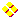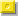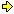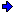HOME plateWON | World!OfNumbersPa!indromic Sumsof the first n 'type of numbers'Sequence ProductsReversal ProductsPythagorean TriplesPalindromes in other BasesPalindromes in ConcatenationsVarious Palindromic Sums

Palindromic Sums

1. Sum of the first n primes is palindromic.

Sequence = 2 + 3 + 5 + 7 + 11 + 13 + ... + z
Index entries in OEIS A038582, A038584 and A038583
Other sources : Puzzle 7

Indexnzpalindromic sum
1122
2235
381977
4769378347285080582
5851087641352888253
612941139241854848458
71461341959253137372273731
863757195640972939156519392
9271988255169169216833383883833386
1053205635104892421327155268786255172
by Jud McCranie

2. Sum of the first n odd primes is palindromic.

Sequence = 3 + 5 + 7 + 11 + 13 + 17 + ... + z
Index entries in OEIS A058845, A058846 and A058847

Indexnzpalindromic sum
1133
2258
3492295115
4542576336
5172103181218
692172133091903    Prime Curios!
71421118637843487    Prime Curios!
812485133853792727297
978653100207337706560773
1019694573192458330398022089303
11560601497137589263888373888362
1290638394183787522781120957675902118
by Patrick De Geest

3. Sum of the first n composites is palindromic.

Sequence = 4 + 6 + 8 + 9 + 10 + 12 + ... + z
Index entries in OEIS A053779, A057959 and A053780
Other sources : Puzzle 89

Indexnzpalindromic sum
1144
22234434
32436505
416721518781
520225827072
622628833633
7144317111254521
8238027853360633
9319037085989895
10395245729145419
114220487310411401
121682718986161101161
132630429509390949093
143761242005795303597
154081345534935424539
1621350123427225127372152
1737652441123277753535777
18192007920741231997671767991
19206108522254802300809080032
20263505728412513754911194573
21346361337289416476856586746
22426858845904019825337335289
231651320617643216146015161510641
2468101132723492702468348558438642
2516642870317626206214693263036239641
2620722436021931114722762189998126722
2740378445042645417986234154745143268
2842127947844487528893856121312165839
29138481348114575275861010599968699950101
30275885958828986621824003657190917563004
31392126437641164906538080970392930790808
326249167674655323594520500472188127400502
by Jeff Heleen

4. Sum of the first n odd composites is palindromic.

Sequence = 9 + 15 + 21 + 25 + 27 + 33 + ... + z
Index entries in OEIS A058848, A058849 and A058850
Other sources : Puzzle 89

Indexnzpalindromic sum
1199
21255404
325991441
4612157227
510835720802
621166373337
7134437252576752
8233963217576757    Prime Curios!
91053926999144666441
10784091913637584554857
1128318167586196520502569
12174874740735333584243424853
13179542341809433776886886773
14238670255401476651010101566
15281908965317899260919190629
1617910160540097417536028948184982063
17192308810642485502914095836722276385904
18282258168862241498818806146443446416088
1977946892701710926635766832347055074323866
by Jeff Heleen [ May 24, 2000 ]
201738101191938023877981331143864040468341133
by Donovan Johnson [ Sep 01, 2012 ]
212563526809355995454633719190811555118091917
by Donovan Johnson [ Sep 01, 2012 ]
222878004326562836534343906041488626884140609
by Chai Wah Wu [ Dec 06, 2019 ]
229797352625321295581541710450905369496350905401
by Chai Wah Wu [ Dec 06, 2019 ]

5. Sum of the first n even composites is palindromic.

Sequence = 4 + 6 + 8 + 10 + 12 + 14 + ... + z
Index entries in OEIS A028553, A058851 and A028554

AldoPalindromic numbers of the form n(n+3).
Main source see Palindromes of the form n(n+3)

The following substitution shows why the sequence equals x * (x + 3)The sequence can be rewritten as 2 * (2 + 3 + 4 + 5 + 6 + 7 + 8 + 9 + ...)
The second term is 'the sum of the natural numbers' minus the starting number 1.
Replace it with the general formula we get 2 * [ (n^2 + n)/2 – 1 ] or [ n^2 + n – 2 ]
Substitute n with (x + 1) we get [ (x + 1)^2 + (x + 1) – 2 ] and work it out.
The sequence evolves from [ x^2 + 2x + 1 + x + 1 – 2 ] to [ x^2 + 3x ]
which finally yields to x * (x + 3) QED

Indexnzpalindromic sum
1144
281888
32858868
4661344554
5881788008
621142445154
729859889698
86711344452254
9212642544526254
10299859988996998
112881457630830333038
122936958740862626268
132999859998899969998
14637011274044058008504
1521220642441445032023054
1621267142534445229592254
1729999859999889999699998
186367761273554405485584504
19212220642444144503764673054
20286141957228408187727277818
21299999859999988999996999998
2294434231888684889178266287198
232134169142683384455467838764554
242886288357725768833066101660338
252999999859999998899999969999998
2621232520642465041445081993739918054
2728905368357810736883552032523025538
2829412732858825465886510885958801568
2929417466958834934086538736763783568
3029999999859999999889999999699999998 = Infinite pattern
Continued atPalindromes of the form n(n+3)

6. Sum of the first n palindromes is palindromic.

Sequence = 1 + 2 + 3 + 4 + 5 + 6 + ... + z
Index entries in OEIS A046486 and A046487

Indexnzpalindromic sum
1111
2223
3336
41233111
51677353
6473837557
7314215122376732
by Patrick De Geest

7. Sum of the first n numbers is palindromic.

Sequence = 1 + 2 + 3 + 4 + 5 + 6 + ... + z
Main source see Palindromic Triangulars

8. Sum of the first n odd numbers is palindromic.

Sequence = 1 + 3 + 5 + 7 + 9 + 11 + ... + z
Main source see Palindromic Squares

9. Sum of the first n even numbers is palindromic.

Sequence = 2 + 4 + 6 + 8 + 10 + 12 + ... + z
Main source see Palindromic Pronic Numbers
```

```

Contributions

Enoch Haga (email) from California, USA.

Jeff Heleen (email) from New Hampshire, USA.

G. L. Honaker, Jr. (email) from Bristol, Virginia, USA.

Jud McCranie (email) from USA.

Carlos Rivera (email) from Monterrey, Nuevo León, México.

```

```

[TOP OF PAGE]

Patrick De Geest - Belgium- Short Bio - Some Pictures